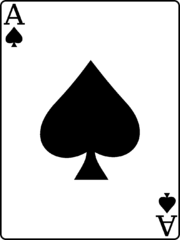# BlackJack II

You're writing a program to play a variety of BlackJack. In general, given two numbers, `a` and `b`, return their sum.

If the sum is greater than `21`, return `0`, unless one of the numbers is `11`. In such a case, the `11` should be 'converted' to a `1` to prevent the sum from being exceeded.

For example, given a `11` and `13` as input, the `11` should be 'converted' into a `1` so the total sum will be `14`.image from wikipedia

### Challenge

Return the sum of two blackjack 'cards', as above.

Alternatively, you can try out Learneroo before signing up.

•#### Matt

Perfect, makes sense. Thanks!

•#### Кирилл Варивода

It's really simple but not boring, great!

•#### Eduardo Bonet

Bug on last entry with javascript for both BlackJack 1 and BlackJack 2: when read the last line I get "7 11", but after I use parseInt my function receives 7 and 1.

•if 11 is converted to 1, last correct output is wrong.

•#### Learneroo

@Borja, it is only converted to 1 if it will otherwise overshoot 21.

•Shame on me :) got totally focused on converting 11 to 1. Thanks for pointing out

•#### Cameron Guyer

Can't figure out how to convert the correct output to 13 and 14 with the correct code. Everything I tried does a compilation error.

•#### thales

Lets say i have 11 and 11. then automaticly it wil return 12. but perhaps i am a weird player of somekind and i want it to be 2. In the casino i can choose this if i want, here i cant?

my code

•#### ani2fun

why compilation fails error ? my code

•#### Prateek

if((a+b)<22 && a!=11 && b!=11){
return a+b;
}
if(a==11 || b==11){

``````            if((a+b)>21){
if(a==11)
a=1;
if(b==11)
b=1;
}

return a+b;
}

return 0;
``````
•#### Dharnish

very simple....
return a+b<21?a+b:(a==11||b==11)?a+b-10:0;

•#### vignesh

return a+b>21?(a==11?b+1:(b==11?a+1:0)):a+b;

•#### Roberto Blanco Axelsson

One way to do it:

int sum = a+b;
int one = 1;

``````    if(sum>21){

if(a == 11){
return one+b;
}
if(b == 11){
return one+a;
}
return 0;
}

return sum;
}
``````
•#### Hanlise

def do_stuff(a, b):
sum1=a+b
if a ==11:
a=1

``````if b == 11:
b=1

else:
return a+b
if sum1 > 21:
return 0
return a+b
``````

This is my code but it's weird. But I got 2 incorrect output